# What Is Electric Current?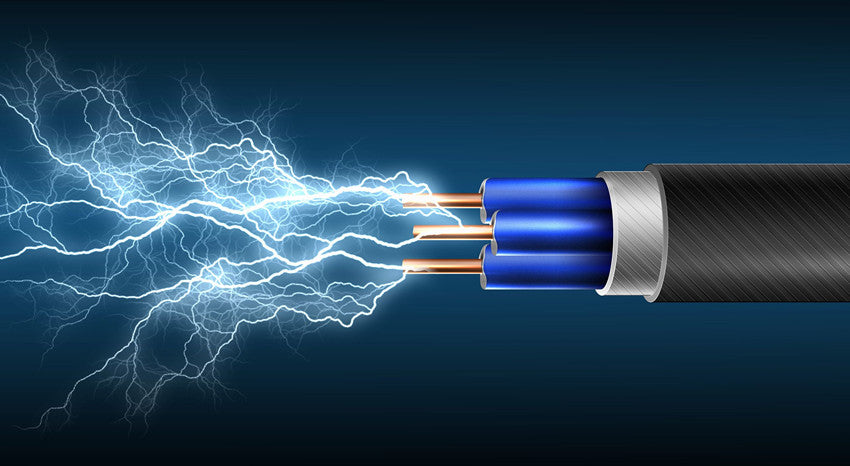Electric current is one of the most basic concepts that exist in electrical and electronic science - electric current is at the core of the science of electricity. Whether it is an electrical heater, a large electrical grid system, a cell phone, a computer, a remote sensor node, or whatever, the concept of electrical current is central to its operation.

### What Is Electric Current?

Electric current is an electric charge in motion. It can take the form of a sudden discharge of static electricity, like a lightning bolt or a spark between your finger and a ground light switch plate. More commonly, though, when we talk about electric current, we mean the more controlled form of electricity from generators, batteries, solar cells, or fuel cells.

Most electric charges are carried by the electrons and protons within atoms. While the proton has a positive charge, the electron has a negative charge. However, protons are mostly immobilized inside atomic nuclei, so the job of transporting charge from one place to another is handled by electrons. Electrons in a conducting material such as a metal are largely free to move from one atom to another along, these conduction bands, which are the highest electron orbits. A Sufficient Electromotive Force (EMF), or voltage generates a charge imbalance that can cause electrons to move through a conductor as an electric current.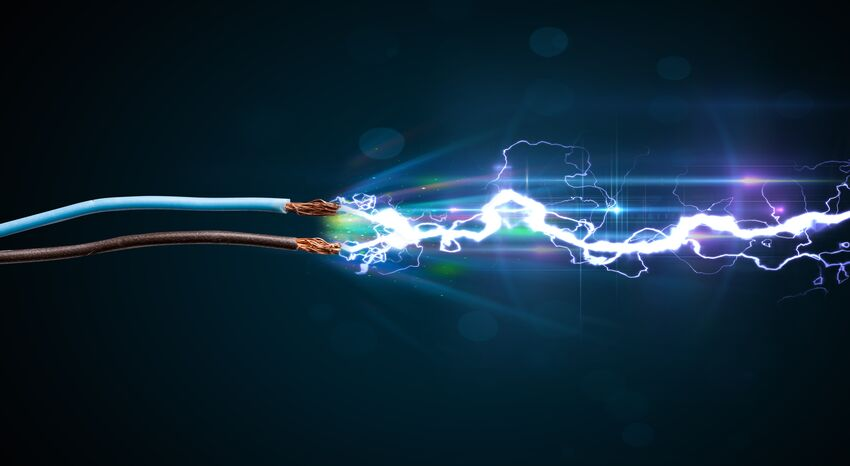### What makes electricity fast?

What makes electricity FAST is the speed at which the electric field interacts with the electron and the speed at which this electric field travels down the wire. For instance, As soon as the electrical circuit is completed, the force that pulls on the electron in the circuit is near-instant, and all the electrons move around roughly at the same time. While an electron leaves the battery from the negative terminal, another one enters the positive terminal on the other side of the circuit; Therefore the current flow can be considered instant.

### The electric current flow

When looking at circuits, there are two types of flow that can demonstrate the flow of electricity; the conventional current and the electron flow. These two move in opposite directions, the conventional current flows moving from positive to negative while electrons flow, being negative charges, moving from negative to positive. While electron flow can be considered more SCIENTIFIC, it can be quite confusing when working with complex components such as logic gates and transistors.

While it is a bit dicey to compare the electric current to the flow of water in a pipe, some similarities that might make it easier to understand. Michael Dobson, a professor of physics at the University of Colorado Boulder, said that we can think of the flow of electrons in a wire as the flow of water in a pipe, The caveat is that in this case the pipe is always full of water. If we open the valve at one end to let water into the pipe, we don't have to wait for the water to make its way all the way to the end of the pipe. We can see the water coming out the other end almost instantaneously as the incoming water pushes the water that's already in the pipe toward the end.

This is what happens to the current in the wire. The conduction electrons already exist in the wire; we just need to push electrons at one end, and they flow almost immediately at the other end.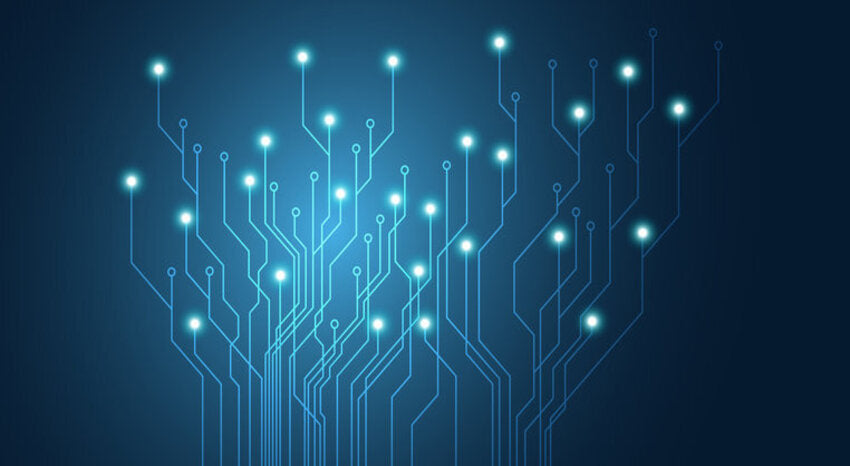### History of Current

Electric currents are named after Andre-Marie Ampere who was considered to be the father of electrodynamics and he has demonstrated the effects of parallel wires and the forces exerted on each depending on the current carried by them. Electric current is measured in amperes, and while multiple definitions exist, an ampere is defined as when one coulomb of charge flows for one second. The old definition for ampere is related to the force experienced on two wires placed apart by a specific distance.

### Current measurement type

Electric current measurement has many types existing, but all fall into one of two categories; analogue and digital. Analogue meters use a coil that deflects upon a current flowing through it, and the amount of deflection is proportional to the current flowing through it. The digital version is instead using a resistor to convert the electric current into a low voltage which is then converted into a digital number.

### Ohm's Law

Out of all equations in electronics, the most commonly used and arguably important formula is V (Voltage) = I (Current) x R (Resistance). It shows how voltage, current, and resistance are all related to each other. However, while this formula is correct, it has not been correctly written as the original equation states.

It is significantly important to understand Ohm's Law because it tells us something about the electric current that makes it different from voltage and resistance; an electric current is the result of a voltage and resistance. （Voltage affects the speed of current flowing, and resistance affects the amount of electric current outputting). Voltage and resistance are characteristics are properties of an object, whereas the electric current is created.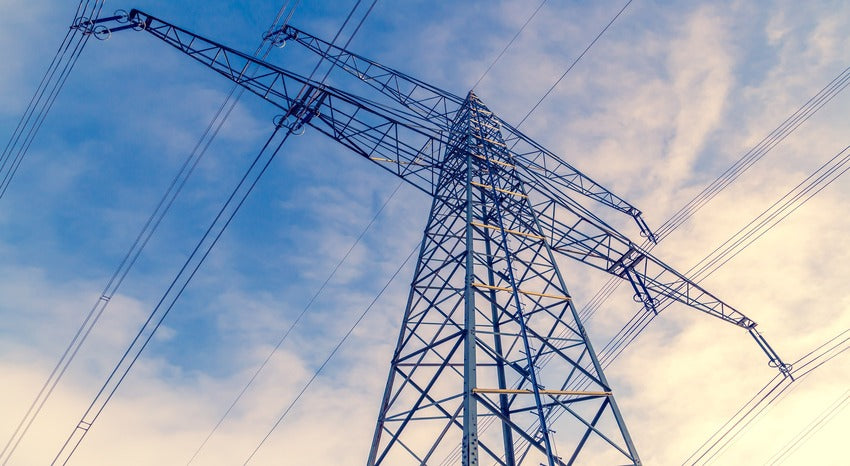### Electric current rules

Electric current follows a set of rules, just like voltage and resistance, depending on parallel and series circuits. Since the same value of electric current rating for a series connection, and the same value of voltage rating for a parallel connection. So, when the electric current flows through components, the electric current flowing through a series circuit is always the same everywhere, and the current has the same value. Thus, if an A of electric current leaves the battery’s positive terminal then an A of current must flow back into the negative terminal.

In parallel, the electric current will split up proportionally depending on the resistance of each path. It is a common myth that electric current always takes the path of least resistance. Instead, the electric current takes ALL paths, but the amount of the current in each path will be determined by the overall resistance. This leads us to the parallel current rule, which states that the sum of the currents entering a node is equal to the sum of the currents leaving the same node. For example, if 3A of electric current goes into a three-way resistor network then the sum of the currents of each resistor network would be 3A.

### The speed of electric current

According to the Hyperphysics website of Georgia State University, the actual speed of electrons in wires is on the order of a few million meters per second, but they do not travel in a straight line down wires. They bounce around nearly at random and only make progress at several millimeters per second. This is called the electron's drift velocity. However, the transmission speed of the signal, when electrons start being pushed out the other end of the wires after we flip the switch, which is nearly the speed of light, is around 300 million meters per second (186,000 miles per second). In the case of Alternating Current, the electric current changes direction 50 or 60 times per second, and most of the electrons never make it out of the wire.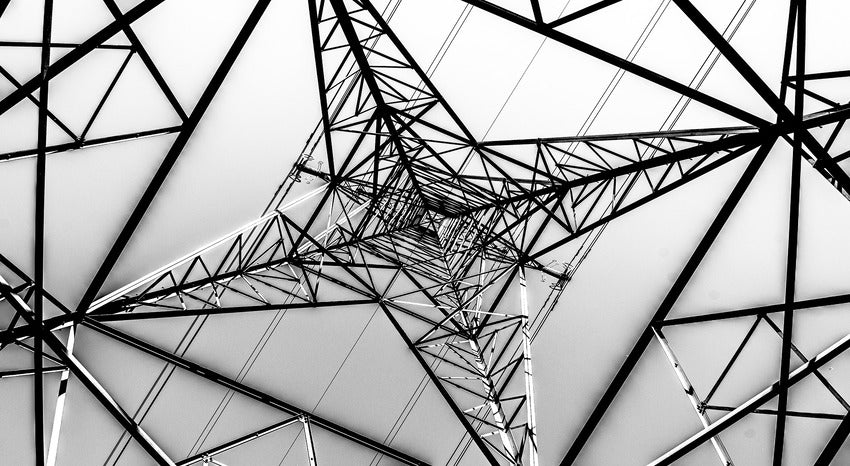### What is an electric current imbalance?

Electric charge imbalances can be created in many ways. The first known method is to generate a static charge by rubbing two different materials together, like rubbing animal fur with a piece of amber. An electric current could then be produced by touching the amber to a body with less charge or to the ground. However, this electric current has a very high voltages, a very low amperages, and lasted for only a fraction of a second, so it could not be made to do any kind of useful work.

### Direct current

The next known method of creating an electric charge imbalance was the electrochemical battery, it is invented in 1800 by the Italian physicist Alessandro Volta for whom the unit for electromotive force, the volt (V) was named. His Voltaic Pile consisted of a stack of alternating zinc and copper plates separated by layers of paper soaked in salt water and generated a steady source of Direct Current (DC). He and other scientists improved and refined his invention over the next few decades. According to the National Museum of American History, batteries attracted the attention of many scientists and inventors, and by the 1840s, batteries were providing electric currents for new electrical devices such as Joseph Henry's electromagnets and Samuel Morse's telegraph.

Other DC power sources include fuel cells, which combine oxygen and hydrogen into water and create electrical energy in the process. The oxygen and the hydrogen could be supplied as pure gasses or from the air and a chemical fuel such as alcohol. Another electrical source of direct current (DC) is photovoltaic or solar cells. In these devices, the photonic energy comes from the sunlight which is absorbed by electrons and converted into electrical energy.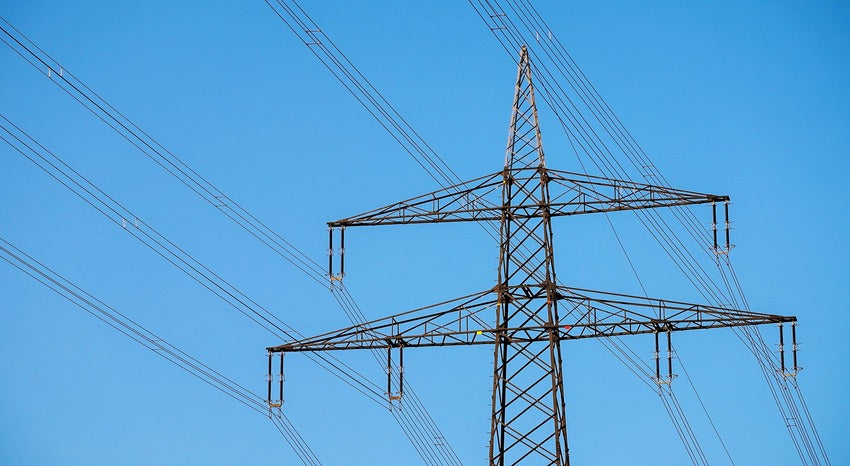### Alternating current

Today, we may all know that most of the electricity we use comes in a form of Alternating Current (AC) that is from the electric power grid. Alternating current (AC) is created by electric generators that operate according to Faraday’s Law of Induction, by which a changing magnetic field can induce an electric current in a conductor. A Generator has rotating coils of a wire that pass through a magnetic field as it turns. As the coils rotate, they switch on and off relative with respect to the magnetic field and generated an electric current that reverses direction every half turn. The electric current can go through a complete forward-and-reverse cycle 60 times each second, or 60 hertz (Hz) (50 Hz in some countries). The generator can be powered by steam turbines heated by coal, natural gas, oil, or a nuclear reactor. Also, it can be powered by wind turbines or water turbines in hydroelectric dams.

The electric current flows from the generator through a series of transformers, where it is stepped up to a much higher voltage for transmission. This is because the diameter of the wires determines the amount of current, or amperage, they can handle without overheating and losing energy, however, the voltage is only limited carry by how well the wire is insulated from the ground. It is interesting to note that the electric current is carried by only one wire instead of two. Both sides of Direct Current (DC) are designated as positive and negative. However, since the polarity of AC changes 60 times per second, both sides of the Alternating Current (AC) are designated as hot and ground. In long-distance power transmission lines, the wires carry the hot end, and the ground end travels through the Earth to complete the circuit.

Since power equals voltage times amperage, you can use a higher voltage to send more power to the line at the same amperage. The high voltage is then stepped down as it is distributed through a network of substations until it reaches the transformer near your home, where it is finally stepped down to 110 V. (In the USA, wall sockets and lights run on 110 V at 60 Hz. In Europe, nearly everything runs on 230 V at 50 Hz.)

Once the current reaches the end of the line, most of the current is used in one of two ways: either to provide heat and light through electrical resistance, or creating mechanical motion through electrical induction. There are a few other applications, such as fluorescent lights and microwave ovens come to mind that operates on different principles, but the lion's share of power goes to devices based on resistance and/or inductance. A hairdryer, for example, uses both at the same time.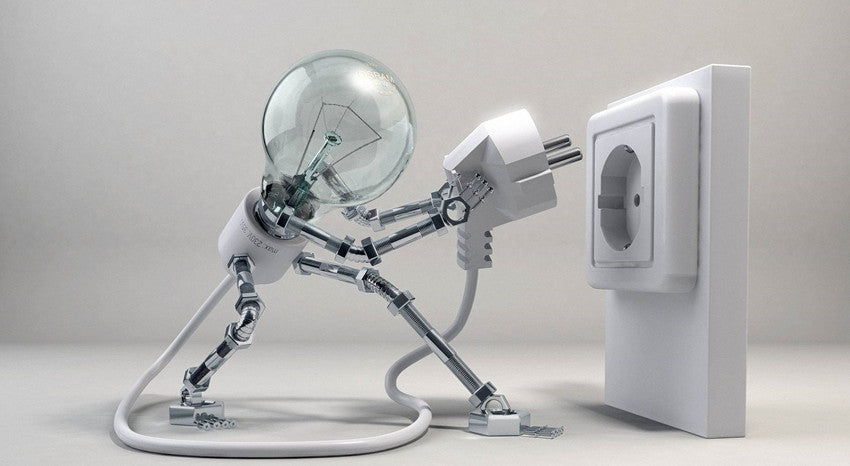### Conclusion

The electric current acts a significantly important role in our lives: it can do work. It can light your home, wash and dry your clothes, power your appliances, and even raise your garage door at the flip of the switch. What is becoming more and more important, though, is the ability of electric current to convey information, especially in the form of binary data. Although the Internet connection to your computer uses only a tiny fraction of the electric current of an electric heater, it is becoming more and more important to modern life.

You have successfully subscribed!
This email has been registered